# LeNet论文解读

### 其他方法

1.例如Gauss-Newton（GN）方法或者Levenberg-Marquardt（LM）方法

1. Quasi-Newton 方法包含Broyden-Fletcher-Goldfarb-Shanno（BFGS） 方法，Limited-storage BFGS（ls-BFGS）算法

1. GN和LM是 $O(N^3)$ 的时间复杂度，N是参数数量
2. QN是 $O(N^2)$ 的时间复杂度
3. ls-BFGS和CG是 $O(N)$ 的复杂度
以上算法的收敛速度取决于选择下降的方向是否准确，而要想准确就要使用全体数据（BG）

error surface就是：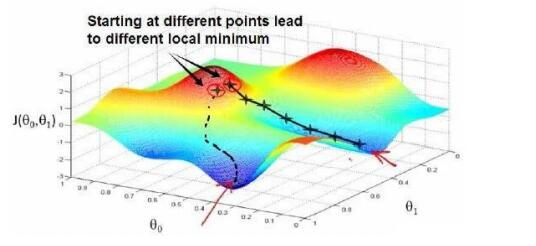## Conditions for Faster Convergence

“Gradient Based Learning Applied to Document Recognition “
LeCun, Y Bottou, L Bengio, Y Haffner, P

### 压缩函数

Squashing function，压缩函数，定义域的范围远远大于值域，这样的函数就是压缩函数，神经网络最常用的就双曲正切函数：

$$f(a)=A tanh(Sa)$$

$$tanh(x)=\frac{e^x-e^{-x}}{e^x+e^{-x}}$$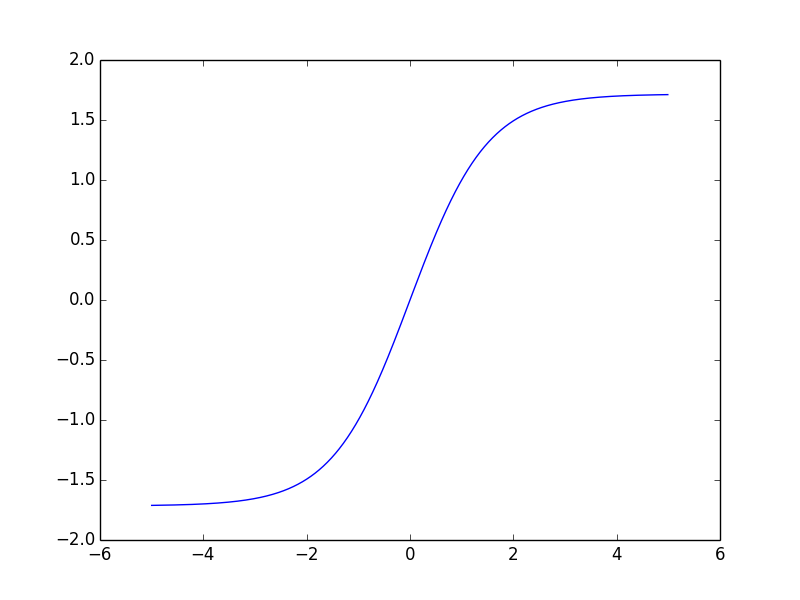### 收敛速度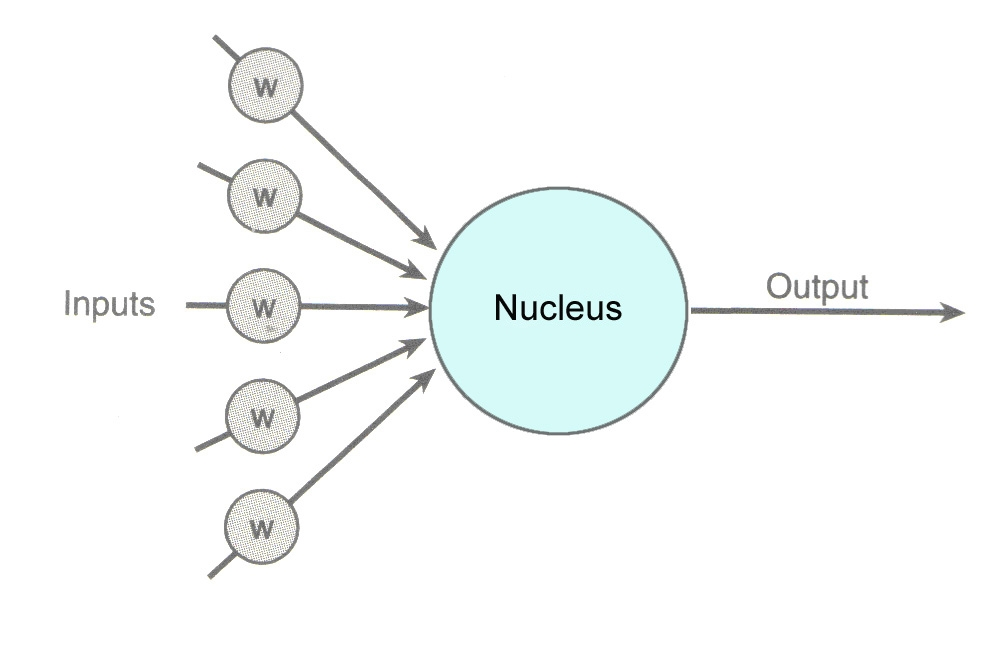$\delta_i^{(l)}=(\sum_{i=1}^{s_{l+1}}W^{(l)}_{ji}\delta^{l+1}_j)f’(z_i^{(l)})$$观察公式可以得出，如果导数过小或者weight过小都会使更新距离变短，这样会使收敛速度变慢。 把激活函数设置成A=1.7159，S=2/3 ，$f(1)=1$，$f(-1)=-1$这么做背后的原因是在正常的处理情况下，压缩的结果会在1附近，这样神经网络的表现将会非常简单。并且f的二次导数在1和-1达到最大值，这使得优化朝向学习最后的阶段（权重收敛的全局最优解）。 观察下图： tanh函数图像：tanh函数一阶导数：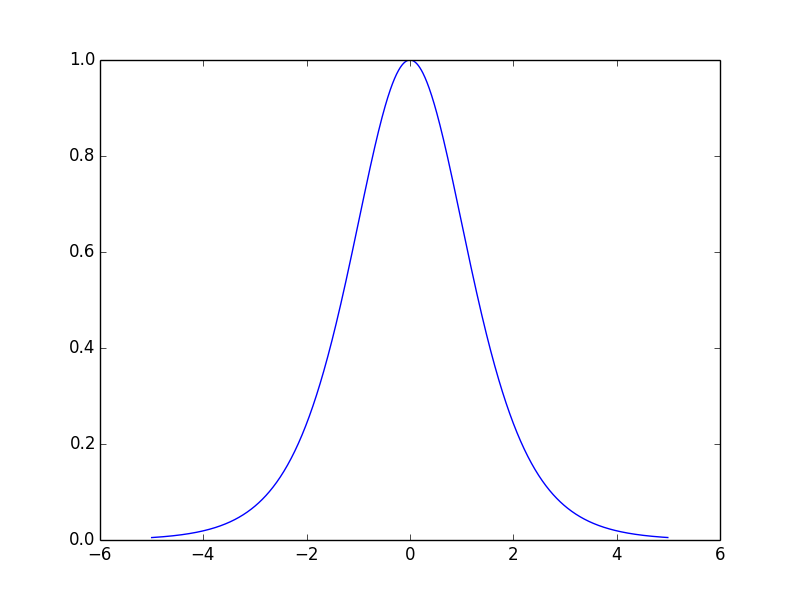tanh函数二阶导数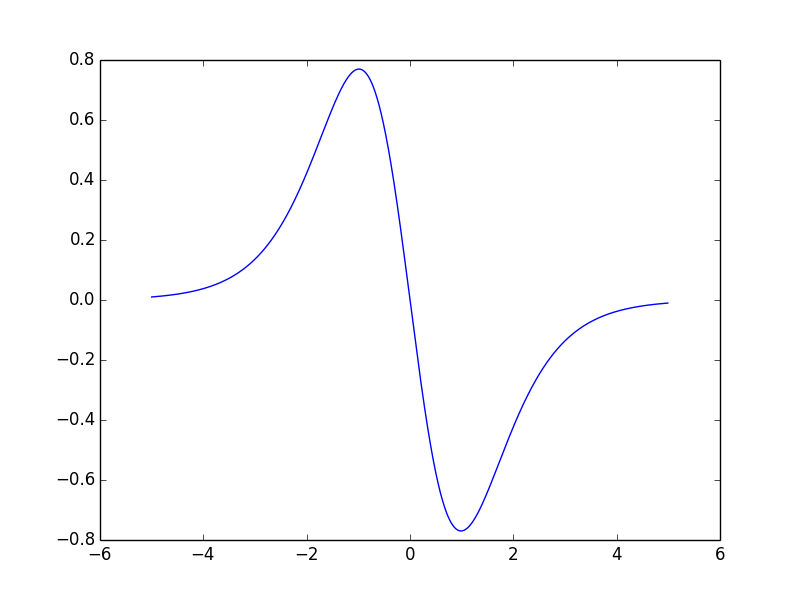二阶导数绝对值在+-1附近最大，二阶导数最大意味着一阶导数最大，这样就意味着梯度（一阶导数）在这附近有最大的变化，这样使得损失函数顺着下降的方向走去。。 ### 参数初始化 特别选择的参数只是能够方便一些，并不影响最终结果 参数初始化：无论是人手工设计还是随机设置，都不太影响结果； 训练开始之前，对所有权重进行均匀分布随机初始化（在$\frac{2.4}{F_i}$和$\frac{-2.4}{F_i}\$ 之间,F为所有连接的总数fan-in，扇入）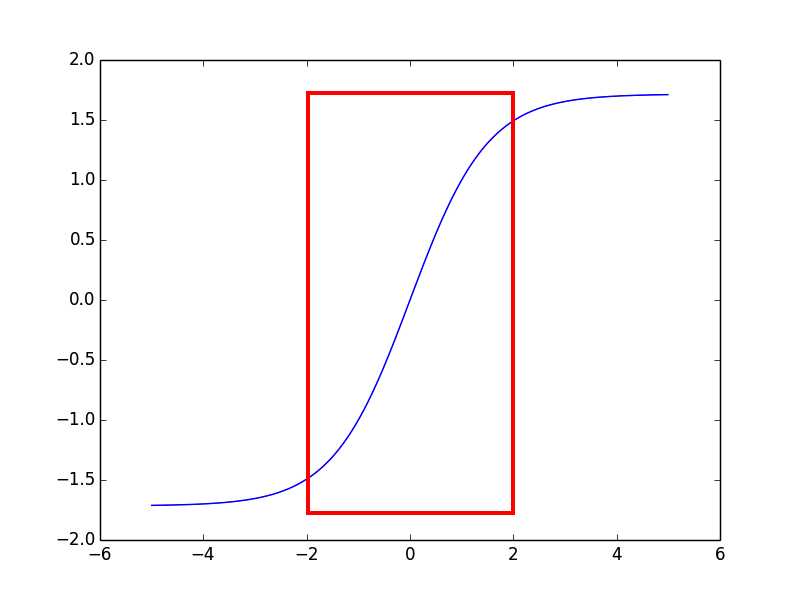0%